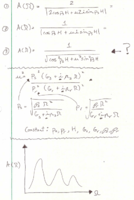# Function with complex numbers

#### AM87

##### New member
Hi,

I'm trying to graph a function that contains imaginary numbers. I may have made a mistake in calculating the modulus of the denominator (step 2 to step 3), or it may be right. Either way, I'm not sure how to get rid of the imaginary numbers, or if they can't be rid of, how to graph the function. I'm trying to use Excel, which I
understand isn't the best program but it's what I've got to work with. Any help would be appreciated. Thanks!#### Jomo

##### Elite Member
Line 3 from line 2: I am assuming that you mean cos(p3H) and not cos(p3)*H. Am I right??
$$\displaystyle A+B \neq \sqrt{A^2+B^2}$$
For example $$\displaystyle 7 = 3+4\neq\sqrt{3^2+4^2}=\sqrt{9+16}= \sqrt{25} = 5$$

Now (a+bi)(a-bi) = a2+ b2. Use this to get the denominator to be real.

Last edited:

#### AM87

##### New member
Line 3 from line 2: I am assuming that you mean cos(p3H) and not cos(p3)*H. Am I right??
$$\displaystyle A+B \neq \sqrt{A^2+B^2}$$
For example $$\displaystyle 7 = 3+4\neq\sqrt{3^2+4^2}=\sqrt{9+16}= \sqrt{25} = 5$$

Now (a+bi)(a-bi) = a2+ b2. Use this to get the denominator to be real.
Yes, what you assumed is right, with cos (PsH). I used the square root of the sum of the squares because those | | I believe are the 'modulus' of what's in the denominator.

| x + iy | = sqrt(x^2 + y^2)

That was my thought process.